# Setting up a DMD: Diffraction effects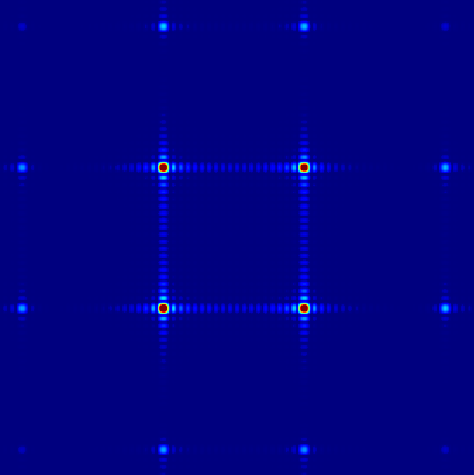I recently acquired a Digital Micromirror Device (DMD) and when I started setting up the experiment, I faced a problem I did not anticipate which is closely related to blazed gratings. Due to the fact that the surface of a DMD is not flat, diffraction orders are shifted compared to the optical axis. This shift depends on the pixel pitch, the wavelength, and the incident angle. A close look at this diffraction phenomenon is important to configure an experimental setup properly. It is even relevant to consider this effect before choosing the appropriate DMD model to buy.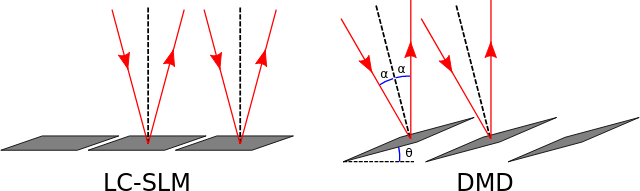Figure 1. Representation of incident and reflected beams with a liquid crystal SLM (left) and a DMD (right).

In a standard diffracting device, such as a flat grating or a liquid crystal SLM, the reflection angle is the opposite of the incident one compared to the normal of the array plane. That implies that the path coming from each element of the device has the same optical path length (Figure 1 left). For a blazed grating, or a DMD with all the pixels in the same state, the elements are tilted with a given angle $$\theta$$. Two consecutive pixels, or elements, now gives rise to a phase difference (Figure 1 right). In the Fourier plane, where we commonly select the zero-th order, all the diffraction orders are shifted compared to the optical axis within the same envelope. In the worst scenario, we can end up with four diffraction orders with approximately the same intensity, each significantly shifted compared to the optical axis. An example is shown in Figure 6. This is almost exactly what I had the first time I set up my DMD while I expected something like in Figure 5.

Another way of seeing this effect is to consider that the diffraction orders (satisfying the grating equation) do not match anymore the angle of the reflected beam.

We first use a 1D model to evaluate the effect of the tilt angle on the diffraction pattern. We then use simple numerical calculations to estimate the profile of the diffraction pattern. The full Python code used in this post is available as an IPython Notebook on my GitHub account: blazing_angle_DMD.ipynb.

### A simple criterion

In a DMD, the axis of rotation of the mirrors is at 45 degrees compared to the axis of the square lattice of the pixels (i.e. the axis of rotation is the diagonal of the square pixels). We consider that we only excite the DMD with a plane wave having a k-vector orthogonal to this axis. This is, in particular, the situation when one rotates the DMD by 45 degrees and illuminates it with a plane wave in the horizontal plane. This makes sense because if one wants to compensate for the diffraction effect in both directions, he has to follow the symmetry of the system. Then, the problem is similar in the $$X$$ and $$Y$$ directions and we can just consider a 1D problem.
For a tilt angle $$\theta$$ in the direction of the diagonal of the pixels, in the $$X$$ and $$Y$$ directions, we consider a tilt angle of $$\theta'=\arctan\left(\tan\left(\theta\right)/\sqrt{2}\right)$$ and an incident angle of $$\alpha'=\arctan\left(\tan\left(\alpha\right)/\sqrt{2}\right)$$.

Ideally, we want to have most of the energy in a central order that corresponds to the optical axis of our system, i.e. that matches the reflection angle. The incident and reflection angles $$\alpha'$$ and $$\beta'$$ have to satisfy the grating equation:

$$\sin\left(\alpha'\right)+\sin\left(\beta'\right)=\frac{m\lambda}{d}$$

This equation gives us the position of the peaks, or diffraction orders, corresponding to integer values of $$m$$. In order to get the maximum intensity, one order has to be at the same angle as the maximum of the envelope. This maximum corresponds to the specular reflection. For a flat grating, this maximum would be at $$\beta' = -\alpha'$$, which coincides with the zero-th order ($$m = 0$$). For a blazed grating with an angle $$\theta'$$, we have:

$$\beta' = 2\theta'-\alpha'$$

Note that $$\theta'$$ can be positive or negative depending on the orientation of the DMD. Rotating it by 180 degrees changes the sign of $$\theta'$$.

In the general case we can calculate the value :

$$m = \frac{2d}{\lambda}\left(\sin(\alpha')+\sin(\beta')\right)$$

If $$m$$ is close to an integer, we are at the blazing condition as in the upper part of Figure 2. If $$m$$ is close to an integer plus 0.5, we are in the situation of the bottom part of Figure 2.

To better quantify if we are close to the blazing condition, we can estimate $$\mu = m \,\%\, 1 -0.5$$. It is equal to 0.5 at the blazing condition and to 0 in the opposite case. I show in Figure 2 $$\mu$$ for $$d = 10.8$$ and $$\lambda=633nm$$ as a function of the incident angle $$\alpha$$.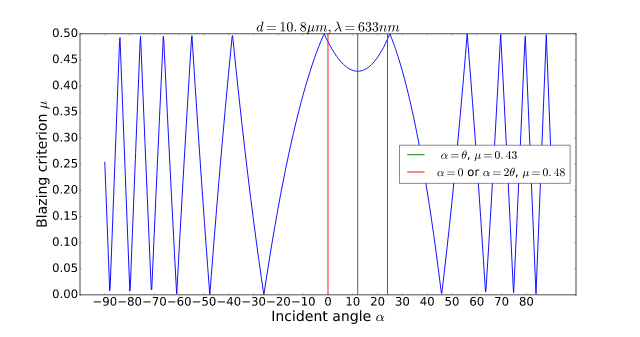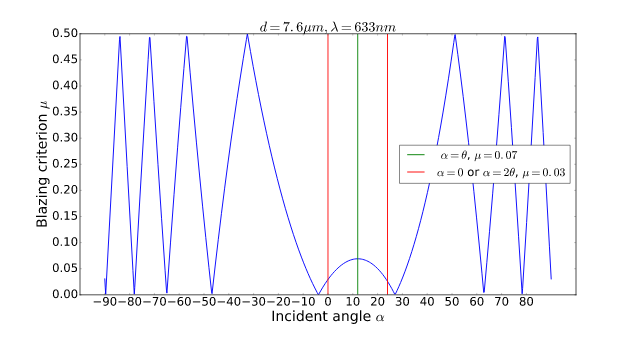Figure 2. Representation of the blazing criterion as a function of the incident angle for $$\lambda = 633nm$$ and for a DMD pith of $$7.6 \mu m$$ (upper figure) and $$10.8 \mu m$$ (bottom figure). Red and green lines correspond to the classical situations with  $$\alpha = \theta$$, the so-called (Littrow configuration) $$\alpha = 0$$ or $$2\theta$$, where either the incident or reflected beam is oriented toward the normal of the DMD plane.

For a given wavelength and a given DMD, so with a fixed pixel pitch, it is possible to retrieve the blazing configuration by tuning the incident angle. However, it may require an inconveniently large angle. This is particularly true when the ratio $$\lambda / d$$ increases, which increases the diffraction angles. In Figure 3, I plotted $$\mu$$ as a function of $$\alpha$$ for $$d = 7.6 \mu m$$ and $$\lambda = 1.55 \mu m$$. We see that the number of oscillations of $$\mu$$ is reduced and since we are close to the worst case, we need to set a very high incident angle to achieve the blazing condition, which is experimentally inconvenient.

Figure 3. Representation of the blazing criterion as a function of the incident angle for $$d = 7.6 \mu m$$ and $$\lambda = 1.55 \mu m$$.

### 2D simulations

To take a look at the diffraction figure of the DMD, we represent the 2D shape of the pixel array. Two neighboring pixels have a phase shift of  $$\frac{2\pi}{\lambda}d\left[\sin(\alpha')+\sin(\beta')\right]$$.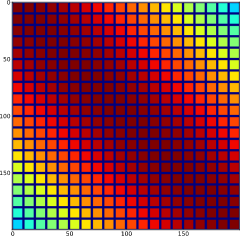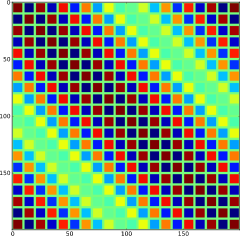Figure 4. Real part of the field contributions of the pixels of the DMD for $$\lambda = 632 nm$$ and $$d = 10.8 \mu m$$ (left) and $$d = 7.6 \mu m$$ (right). For $$d = 10.8 \mu m$$, we are close to the blazing condition and the phase of the pixel contributions is almost flat.

We then display the 2D Fourier transform. To better see the diffraction order, the square root of the intensity is plotted. The diffraction patterns for the configurations in study are displayed in Figure 5 and Figure 6.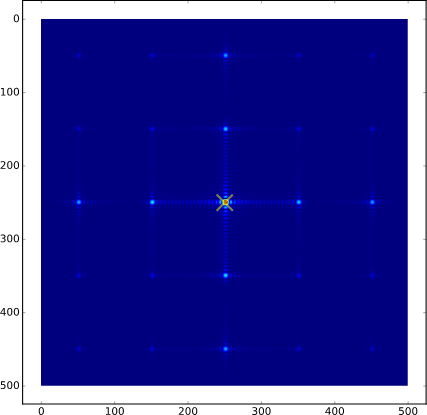Figure 5. Diffraction pattern of an "all-on" DMD array for $$\lambda = 632 nm$$ and $$d = 10.8 \mu m$$. To better see the higher orders, I represented the square root of the intensity. The yellow cross indicates the angle of the optical axis.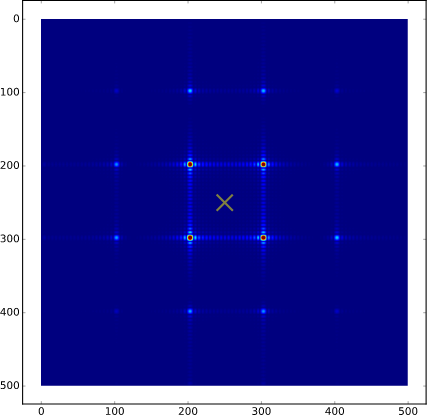Figure 6. Diffraction pattern of an "all-on" DMD array for $$\lambda = 632 nm$$ and $$d = 7.6 \mu m$$. To better see the higher orders, I represented the square root of the intensity. The yellow cross indicates the angle of the optical axis.

The full IPython code is available here: blazing_angle_DMD.ipynb

Created by sebastien.popoff on 29/10/2016